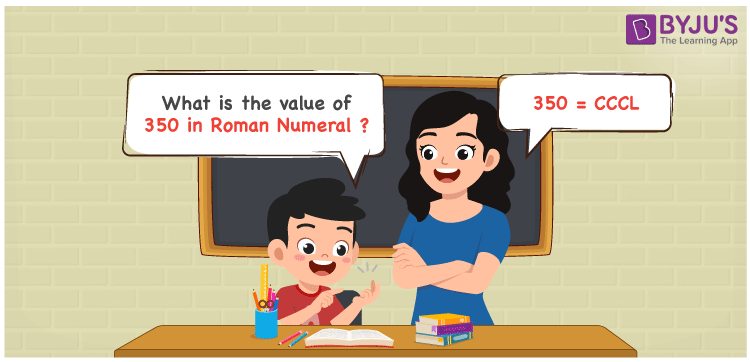# 350 in Roman Numerals

350 in Roman Numerals is CCCL. Any Roman numeral uses only the 7 primary symbols which are I, V, X, L, C, D, and M. Here C represents 100. CC represents the number 350. What are Roman numerals? can be referred for more details. The details on how to write 350 is given in the next section of this article.

 Number Roman Numeral 350 CCCL

## How to Write 350 in Roman Numerals?To convert 350 in Roman Numerals, we need to represent 350 as the sum of the fundamental symbols. 350 can be broken down as the sum of 300 and 50. But we do not have a primary symbol for 300. Hence it needs to be represented as sum of 3 hundred. And L is the symbol for 50. So 350 will be 100 + 100 + 100 + 50.

350 = 100 + 100 + 100 + 50

350 = C + C + C + L

350 = CCCL

## Video Lesson on Roman Numerals## Frequently Asked Questions on 350 in Roman Numerals

Q1

### How to write the number 350 in Roman Numerals?

350 in Roman Numerals is written as CCCL.
Q2

### Does CCCL represent Roman Numerals?

Yes. CCCL represents Roman Numerals and it is the number 350
Q3

### How to represent CCCL in the International number system?

The Roman Numerals CCCL is represented as 350 in the International number system.